Published

# MEGR 3171: Tachometer and Resultant Temperature Detector

This device measures the rotational speed of fans and records the difference of temperatures due to variating speeds said fans.

IntermediateShowcase (no instructions)25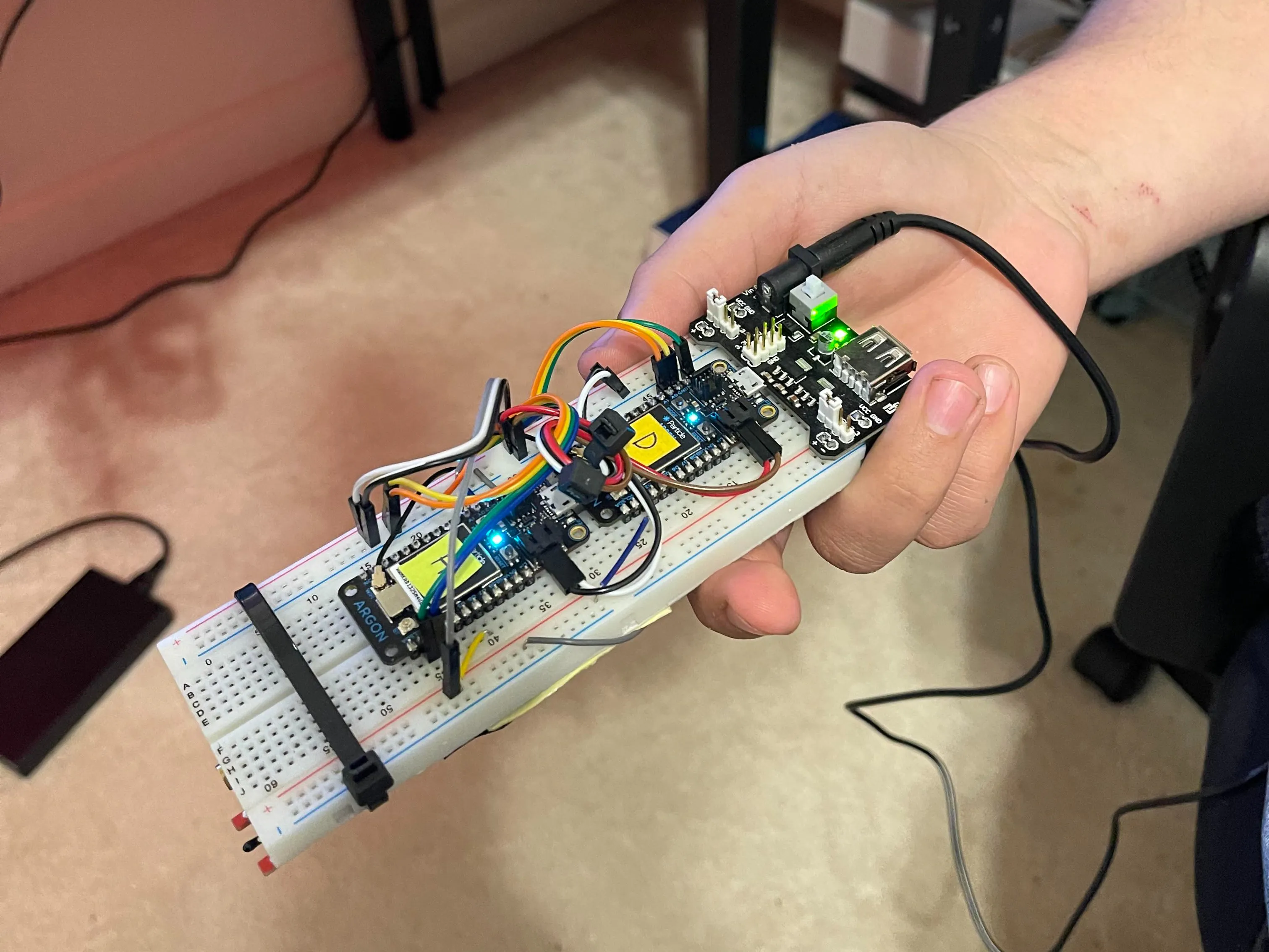## Things used in this project

### Hardware components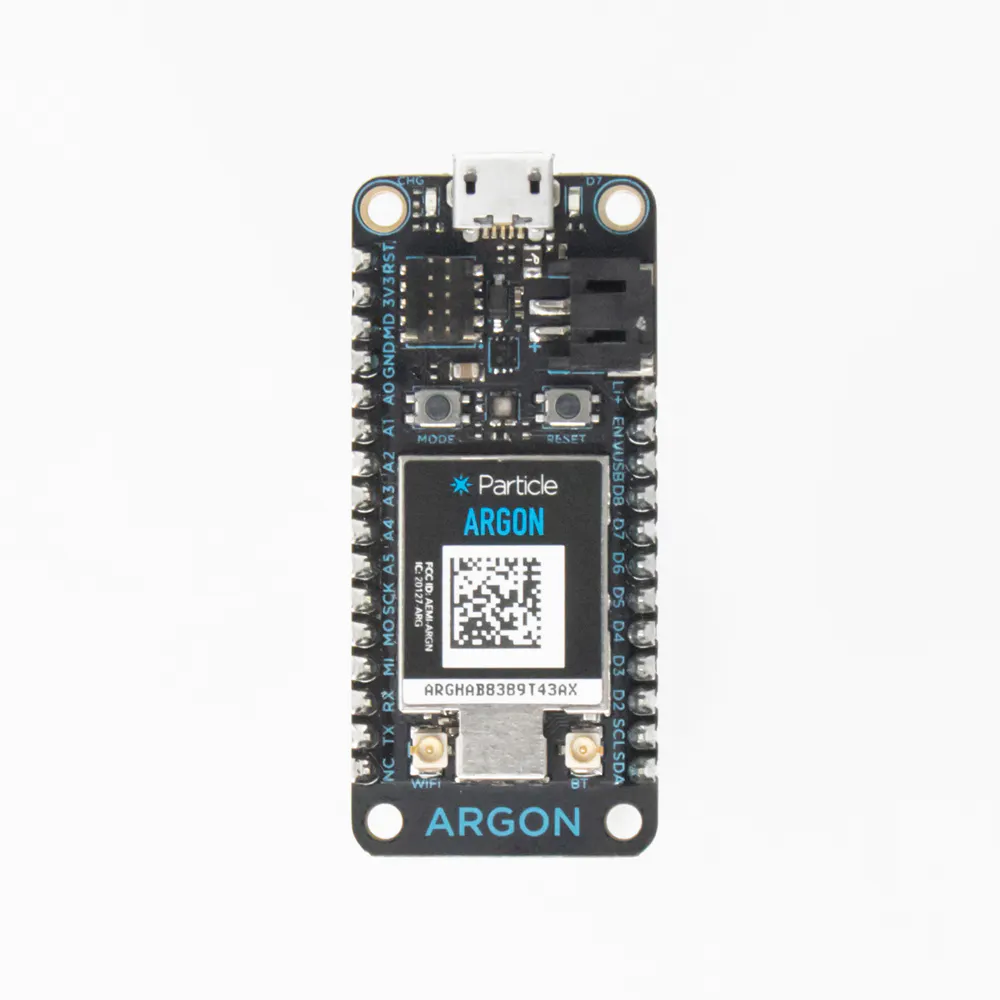Particle Argon
×3
 Elegoo Digital Thermistor
×1
 Elegoo Avoidance Sensor
×1Jumper wires (generic)
×8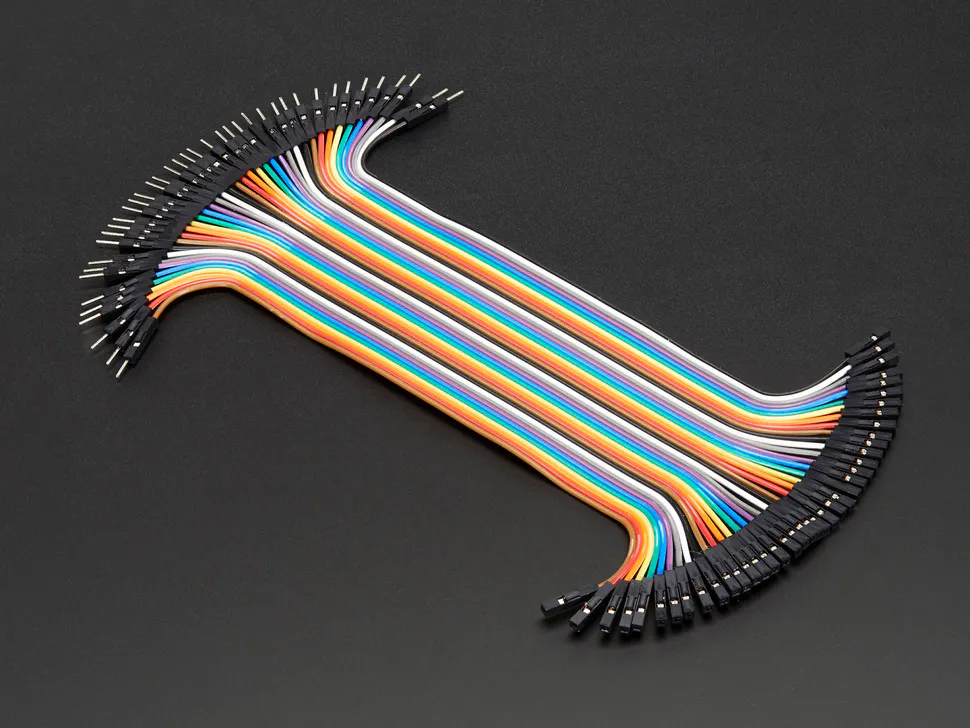Male/Female Jumper Wires
×14
 Elegoo Button
×1Resistor 1k ohm
×1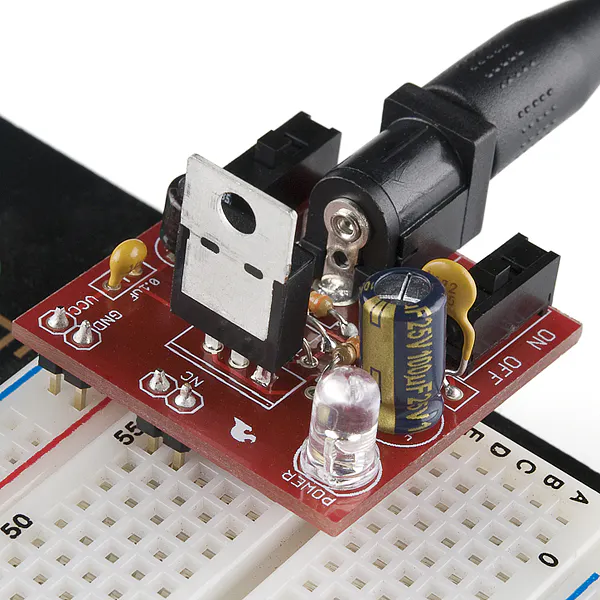×1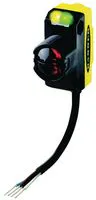Laser Emitter, Small Spot
×1×1

### Software apps and online servicesThingSpeak API https://thingspeak.com/channels/1709769

### Hand tools and fabrication machines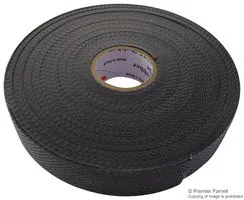Tape, Electrical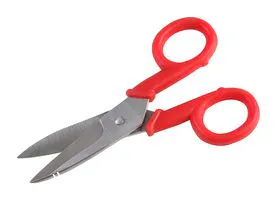Scissor, Electrician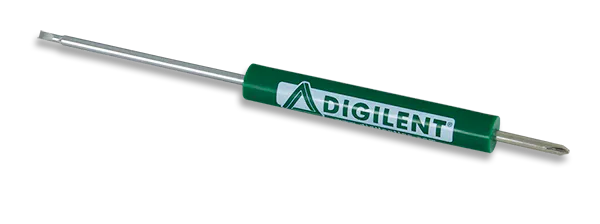Digilent Screwdriver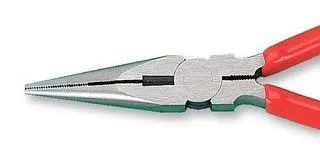Plier, Long Nose

## Schematics

### Temp vs Time

This is our plot for the temperature over time. As a result of the cold air produced by the fan you can see how as the fan was turned on the temperature began to drop over time.

https://thingspeak.com/channels/1709769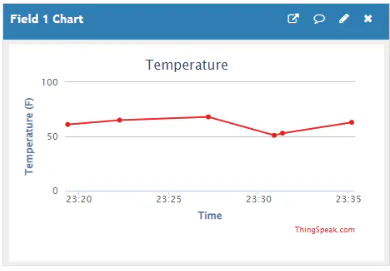### RPM

Using an IR avoidance sensor to detect the revolutions of fan blades over a period of time the RPM can be calculated for the blades.

https://thingspeak.com/channels/1709769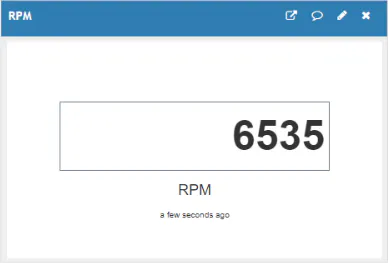### Wiring Diagram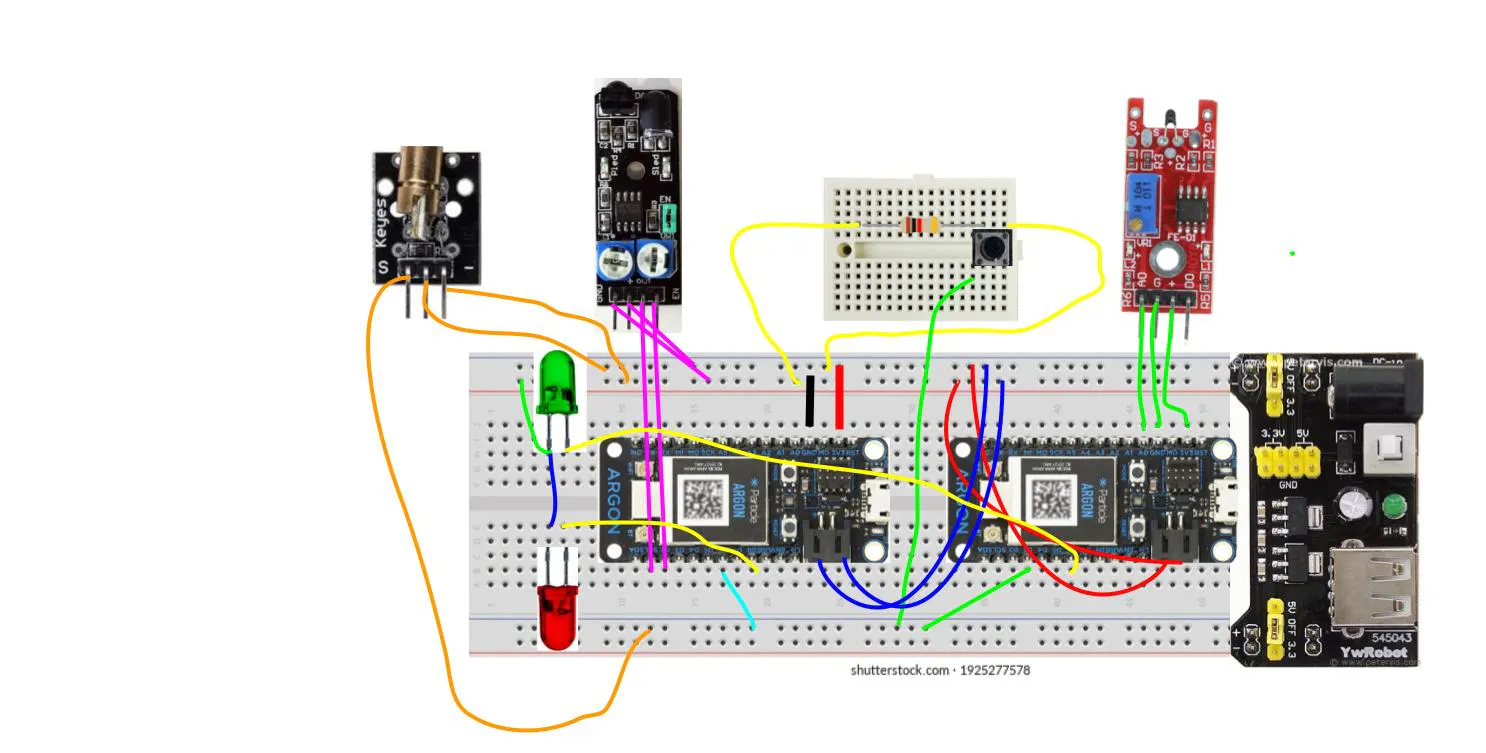### Flow chart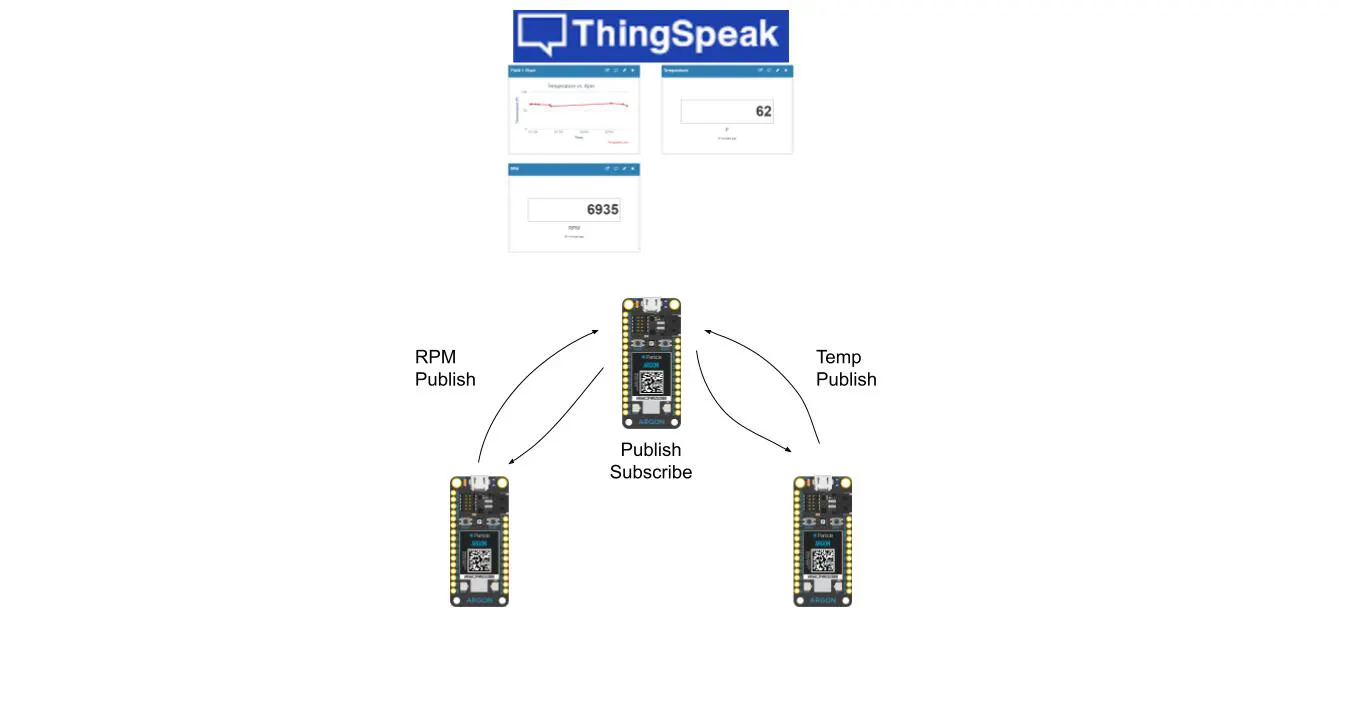## Code

### Subscribe

C/C++
```float Temperature = 0.0000;
float RPM_Recorded = 0.0000;
int tempsuc = 0;
int RPMsuc = 0;

void temper(const char *event, const char *data )
{
int val = atoi(data);
Temperature = val;
Particle.publish("Temperature", String(Temperature));
}

void RPMhandle(const char *event, const char *data )
{
int val = atoi(data);
RPM_Recorded = val;
Particle.publish("RPM", String(RPM_Recorded));
delay(15000);
}

void TempRespHandle(const char *event, const char *data )
{
int val = atoi(data);
tempsuc = val;
Particle.publish("temp_recieved", String(tempsuc));
}

void RPMRespHandle(const char *event, const char *data )
{
int val = atoi(data);
RPMsuc = val;
Particle.publish("RPM_recieved", String(RPMsuc));
}

void setup()
{
Particle.subscribe("TempTherm", temper);
Particle.subscribe("RPM", RPMhandle);
Particle.subscribe("hook-response/Temperature", TempRespHandle);
Particle.subscribe("hook-response/RPM", RPMRespHandle);
}
```

### Tach

C/C++
```int spokes = 3.0000; //Temporary spoke number user input
int ledPin = D7; //board led
int detectorPin = D1; //ir detector pin
int enablePin = D2; //ir enable pin
int triggerPin = D6; //trigger for recording data
int changeSpoke(String command);
int RPMrecievedled = D8;
float rpm = 0.0000;
float count = 0.0000;
unsigned long timeend;
unsigned long timeendcount;
unsigned long timestart = 0;
unsigned long timestartcount = 0;
unsigned long timemilsec;
unsigned long timedelay;
unsigned long timedelayval;
bool object = true;
void setup()
{
pinMode(ledPin, OUTPUT);
pinMode(detectorPin, INPUT);
pinMode(enablePin, OUTPUT);
pinMode(triggerPin, INPUT);
pinMode(RPMrecievedled, OUTPUT);
digitalWrite(enablePin, HIGH);
Particle.function("Spokes", changeSpoke);
Particle.subscribe("RPM_recieved", RPMledHandle);
}
void loop()
{
unsigned long timeend = millis();
unsigned long timeendcount = millis();
if (timeend - timestart >= 1 )
{
timestart = timeend;
if (digitalRead(triggerPin) == HIGH) // Pressed
{
timemilsec++;
}
else
{
//nothing
}
}
if (timeendcount - timestartcount >= 1 )
{
timestartcount = timeendcount;
if (digitalRead(triggerPin) == HIGH) // Pressed
{
timedelay++;
}
else
{
//nothing
}
}
if(trigger == LOW)
{

float revolutions = count/spokes;
float timemin = timemilsec/60000.0000;
float timedisp = timemilsec;
float timesensitivity = timedelay;
rpm = revolutions/(timemilsec/60000.0000);
Particle.publish("Time (Minutes)", String(timemin));
Particle.publish("Revolutions", String(revolutions));
Particle.publish("Spokes", String(spokes));
//Particle.publish("Temp", String(temp));
//Particle.publish("timedelay", String(timesensitivity));
//Particle.publish("timerecord", String(timedisp));
Particle.publish("RPM", String(rpm));
//Particle.publish("RPM", String(rpm));
//Particle.publish("RPM", String(rpm));
//Particle.publish("RPM", String(rpm));
timemilsec = 0.0000;
count = 0.0000;
}
else
{
if(val == LOW)
{
if(timedelay-timedelayval >= 1)
{
if (object==false)
{
count++;
digitalWrite(ledPin,HIGH);
object=true;
timedelayval = timedelay;
}
else
{
//nothing
}
}
else
{
//nothing
}
}
else
{
if(timedelay-timedelayval >= 1)
{
if (object==true)
{
digitalWrite(ledPin,LOW);
object=false;
}
else
{
//nothing
}
}
else
{
//nothing
}
}
}
}
int changeSpoke(String command)
{
if(command == "1")
{
spokes = 1.0000;
return 1;
}
else if (command == "2")
{
spokes = 2.0000;
return 1;
}
else if (command == "3")
{
spokes = 3.0000;
return 1;
}
else if (command == "4")
{
spokes = 4.0000;
return 1;
}
else if (command == "5")
{
spokes = 5.0000;
return 1;
}
else if (command == "6")
{
spokes = 6.0000;
return 1;
}
else if (command == "7")
{
spokes = 7.0000;
return 1;
}
else if (command == "8")
{
spokes = 8.0000;
return 1;
}
else if (command == "9")
{
spokes = 9.0000;
return 1;
}
else if (command == "10")
{
spokes = 10.0000;
return 1;
}
else if (command == "11")
{
spokes = 11.0000;
return 1;
}
else if (command == "12")
{
spokes = 12.0000;
return 1;
}
else if (command == "13")
{
spokes = 13.0000;
return 1;
}
else if (command == "14")
{
spokes = 14.0000;
return 1;
}
else if (command == "15")
{
spokes = 15.0000;
return 1;
}
else if (command == "16")
{
spokes = 16.0000;
return 1;
}
else if (command == "17")
{
spokes = 17.0000;
return 1;
}
else if (command == "18")
{
spokes = 18.0000;
return 1;
}
else if (command == "19")
{
spokes = 19.0000;
return 1;
}
else if (command == "20")
{
spokes = 20.0000;
return 1;
}
else if (command == "21")
{
spokes = 21.0000;
return 1;
}
else if (command == "22")
{
spokes = 22.0000;
return 1;
}
else if (command == "23")
{
spokes = 23.0000;
return 1;
}
else if (command == "24")
{
spokes = 24.0000;
return 1;
}
else if (command == "25")
{
spokes = 25.0000;
return 1;
}
else if (command == "26")
{
spokes = 26.0000;
return 1;
}
else if (command == "27")
{
spokes = 27.0000;
return 1;
}
else if (command == "28")
{
spokes = 28.0000;
return 1;
}
else if (command == "29")
{
spokes = 29.0000;
return 1;
}
else if (command == "30")
{
spokes = 30.0000;
return 1;
}
else if (command == "31")
{
spokes = 31.0000;
return 1;
}
else if (command == "32")
{
spokes = 32.0000;
return 1;
}
else if (command == "33")
{
spokes = 33.0000;
return 1;
}
else if (command == "34")
{
spokes = 34.0000;
return 1;
}
else if (command == "35")
{
spokes = 35.0000;
return 1;
}
else if (command == "36")
{
spokes = 36.0000;
return 1;
}
else if (command == "37")
{
spokes = 37.0000;
return 1;
}
else if (command == "38")
{
spokes = 38.0000;
return 1;
}
else if (command == "39")
{
spokes = 39.0000;
return 1;
}
else if (command == "40")
{
spokes = 40.0000;
return 1;
}
else if (command == "41")
{
spokes = 41.0000;
return 1;
}
else if (command == "42")
{
spokes = 42.0000;
return 1;
}
else if (command == "43")
{
spokes = 43.0000;
return 1;
}
else if (command == "44")
{
spokes = 44.0000;
return 1;
}
else if (command == "45")
{
spokes = 45.0000;
return 1;
}
else if (command == "46")
{
spokes = 46.0000;
return 1;
}
else if (command == "47")
{
spokes = 47.0000;
return 1;
}
else if (command == "48")
{
spokes = 48.0000;
return 1;
}
else if (command == "49")
{
spokes = 49.0000;
return 1;
}
else if (command == "50")
{
spokes = 50.0000;
return 1;
}
else
{
return -1;
}
}
void RPMledHandle(const char *event, const char *data )
{
digitalWrite(RPMrecievedled, HIGH);
delay(100);
digitalWrite(RPMrecievedled, LOW);
delay(100);
digitalWrite(RPMrecievedled, HIGH);
delay(100);
digitalWrite(RPMrecievedled, LOW);
delay(100);
digitalWrite(RPMrecievedled, HIGH);
delay(100);
digitalWrite(RPMrecievedled, LOW);
}
```

### Thermistor

C/C++
```int analogvalue = 0;
double temp = 0;
int tims = 0;
int detect = D2;
int temprecievedled = D6;
void setup()
{
Particle.variable("analogvalue", analogvalue);
Particle.variable("temp", temp);
Particle.variable("time", tims);

Particle.subscribe("temp_recieved", templedHandle);

pinMode(A0, INPUT);
pinMode(D2, INPUT);
pinMode(D6, OUTPUT);
tims = 0;
}

void loop()
{
if(trigger == HIGH)
{
delay(7500);
// Read the analog value of the sensor (TMP36)
temp = (analogvalue*-.08099688473520249+151.99688473520249); //To be accurate
//temp = 69;
Particle.publish("TempTherm", String(temp));
delay(7500);
temp = 0;
tims = tims + 1000;
if(tims > 5000)
{
tims = 0;
}
}
else
{

}
}
void templedHandle(const char *event, const char *data )
{
digitalWrite(temprecievedled, HIGH);
delay(100);
digitalWrite(temprecievedled, LOW);
delay(100);
digitalWrite(temprecievedled, HIGH);
delay(100);
digitalWrite(temprecievedled, LOW);
delay(100);
digitalWrite(temprecievedled, HIGH);
delay(100);
digitalWrite(temprecievedled, LOW);
}
```

## Credits

### Dwood40

0 projects • 0 followers

### Hunter Mathis

0 projects • 0 followers

### zduvall31

0 projects • 0 followers